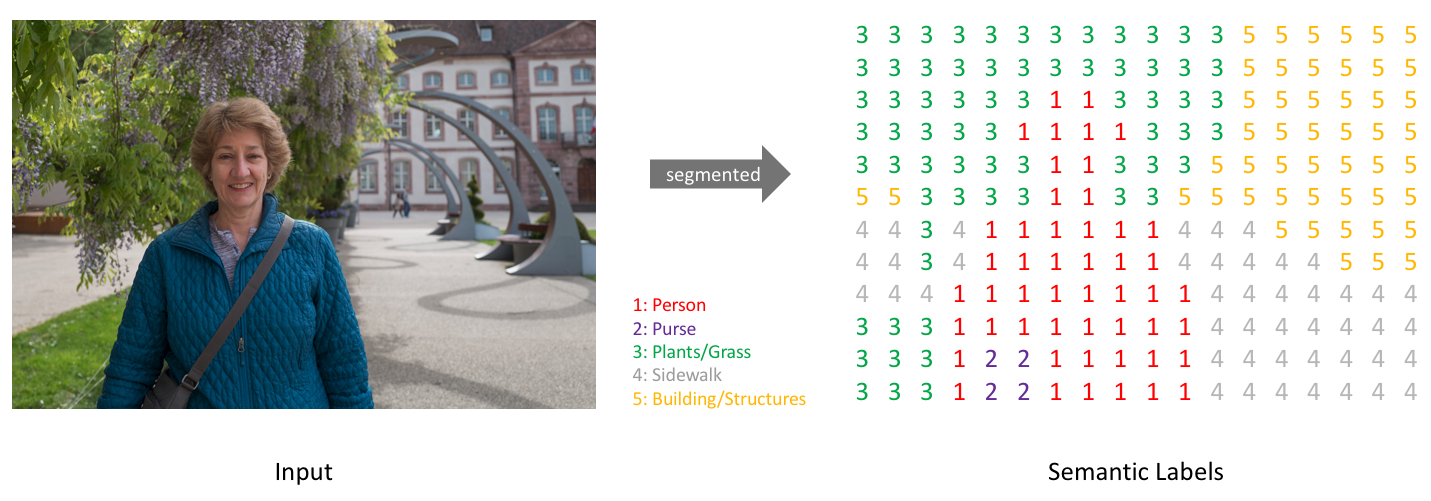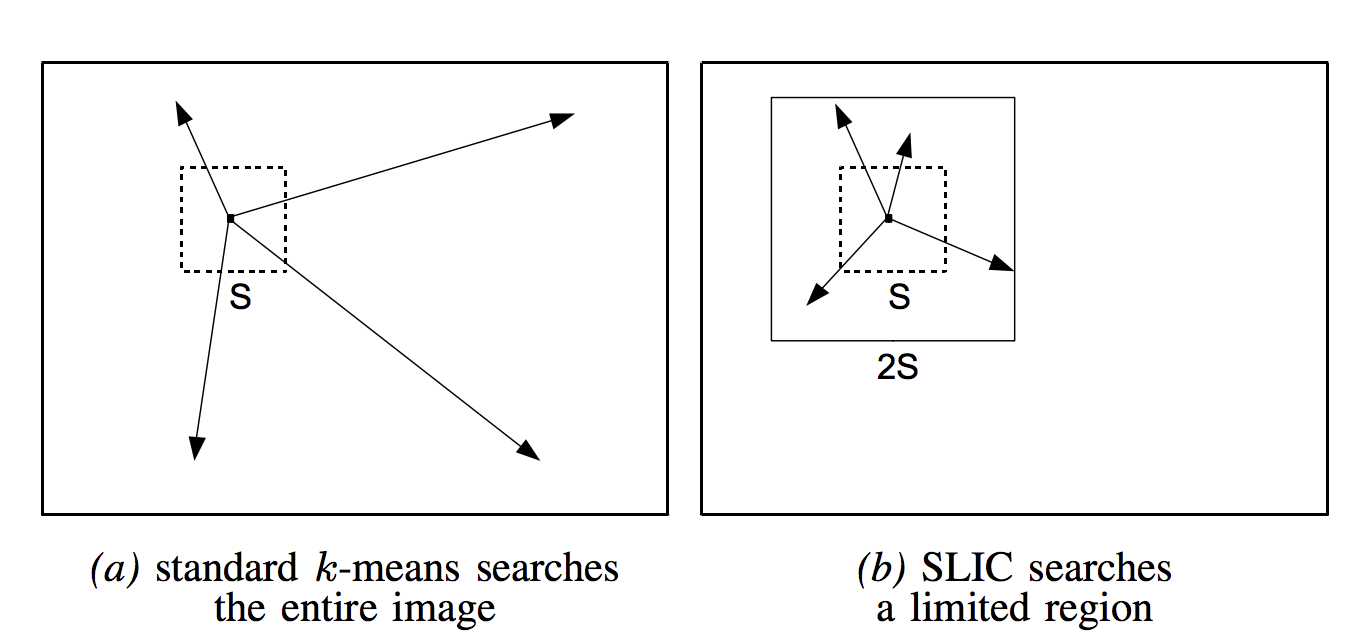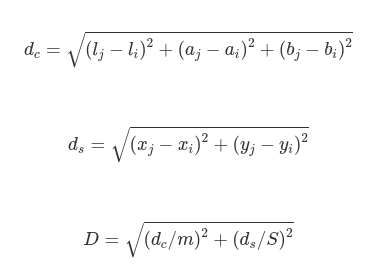## Motivation

Image segmentation plays a great role in order to realize which objects construct an image. In order to reach this goal, people have invented a lot of strategies – one of which is superpixel.

SLIC is a well-known algorithm which runs in linear time complexity , and has been implemented in various image processing libraries including OpenCV and scikit-image. However, JulaImages has not implemented SLIC yet, though this Julia image processing package contains Felzenswalb and quick shift. Therefore, I want to implement SLIC in Julia to let me familiar with SLIC algorithm but also Julia.

## Image segmentation

Image segmentation is a process to classify which cluster each pixel should belong to. The goal of this is to let image more representative and easier for us to analyze. So far, people have invented a lot of techniques according to each characteristic of each object such as color, texture, and intensity, etc.A clear representation of image segmentation. Image source: jeremyjordan.me

## What’s Superpixel?

We use the properties of a pixel to classify which object it should belongs to. However, let me give you a question: is it proper to determine a range of an object in such small scale? Seems not. In 2003, Xiaofeng Ren and Jitendra Malik discussed about this problem and proposed superpixel.  The intuition of superpixel is pretty simple: rather than determine each pixel, we can group pixels with akin properties into a larger one – called superpixel – for further analyze. In this way, we not only get more meaningful regions but also improve computational efficiency. 

## SLIC (Simple Linear Iterative Clustering)

We can use some strategies to cluster pixels with similar properties into a superpixel such as graph theorem, gradient decent, or even machine learning methods. As the most well-known one of using machine learning method, SLIC comes to the place. The intuition of SLIC is pretty naive: we can set some seeds to cluster the surrounding pixels to form superpixels.

You will ask a problem: “It seems pretty straightforward. So what’s special of SLIC?” Rather than search all the pixels like normal K-means, SLIC uses a modified K-means which restricts the searching area into a certain space. To my opinion, SLIC adopts this way because each pixel surrounding to a center should have similar properties.A comparison between normal K-means and SLIC modified K-means. Taken from 

### Algorithm

SLIC just requires two input parameters:

1. K: number of superpixels.
2. m: compactness factor to let superpixel be more compact or not.

And the simplified distance calculation is shown as follows:Where S = sqrt(N/K) (N represents the number of pixels.)

The following lines describe the algorithm of SLIC.

``````/∗ Initialization ∗/
Initialize cluster centers Ck = [lk , ak , bk , xk , yk ]T by sampling pixels at regular grid steps S.
Move cluster centers to the lowest gradient position in a 3 × 3 neighborhood.
Set label l(i) = −1 for each pixel i. Set distance d(i) = ∞ for each pixel i.

repeat
/∗ Assignment ∗/
for each cluster center Ck do
for each pixel i in a 2S × 2S region around Ck do
Compute the distance D between Ck and i.
if D < d(i) then
set d(i) = D
set l(i) = k
end if
end for
end for

/∗ Update ∗/
Compute new cluster centers. Compute residual error E.
until E ≤ threshold
``````

## Why use Julia?

As a new-born language, Julia grasps my sight because it is fast, dynamic, and general. Though SLIC has been implemented in various image processing libraries such as OpenCV and scikit-image, there are no any implementations in pure Julia, which seems a pity that we don’t use the benefits of this programming language.

## Just Show Me The Code!

Because it is lengthy, you can view the code here. Feel free to ask any questions if you cannot figure out :)

## Recap

In this post, I introduce the concept of image segmentation as well as the history of superpixel. Next, I make an introduction of SLIC superpixel and the algorithm. Last, I express why I want to implement SLIC in pure Julia and leave the link of my implementation.

For the future aspect, I will work on enforce connectivity after we generate the superpixel.